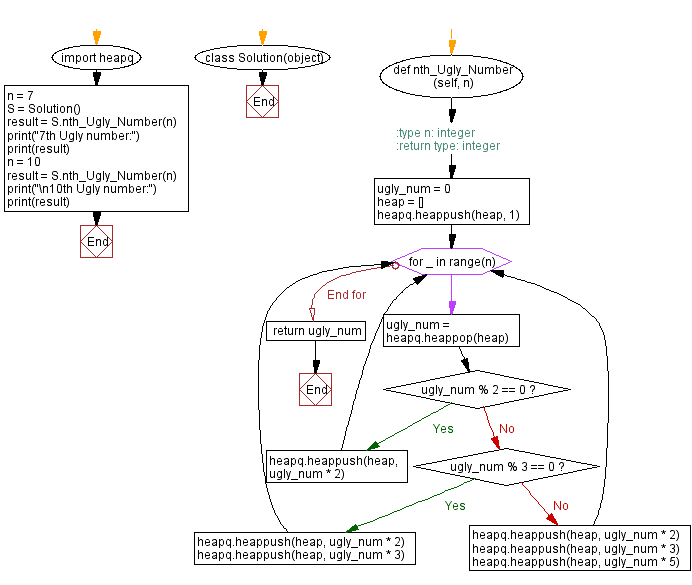﻿ Python heap queue algorithm: Find the nth ugly number using Heap queue algorithm - w3resource

# Python: Find the nth ugly number using Heap queue algorithm

## Python heap queue algorithm: Exercise-18 with Solution

Write a Python program to find the nth ugly number using the heap queue algorithm.

Ugly numbers are positive numbers whose only prime factors are 2, 3 or 5. The sequence 1, 2, 3, 4, 5, 6, 8, 9, 10, 12, ... shows the first 10 ugly numbers.
Note: 1 is typically treated as an ugly number.

Sample Solution:

Python Code:

``````import heapq
class Solution(object):
#:type n: integer
#:return type: integer
def nth_Ugly_Number(self, n):
ugly_num = 0
heap = []
heapq.heappush(heap, 1)
for _ in range(n):
ugly_num = heapq.heappop(heap)
if ugly_num % 2 == 0:
heapq.heappush(heap, ugly_num * 2)
elif ugly_num % 3 == 0:
heapq.heappush(heap, ugly_num * 2)
heapq.heappush(heap, ugly_num * 3)
else:
heapq.heappush(heap, ugly_num * 2)
heapq.heappush(heap, ugly_num * 3)
heapq.heappush(heap, ugly_num * 5)
return ugly_num
n = 7
S = Solution()
result = S.nth_Ugly_Number(n)
print("7th Ugly number:")
print(result)
n = 10
result = S.nth_Ugly_Number(n)
print("\n10th Ugly number:")
print(result)
```
```

Sample Output:

```7th Ugly number:
8
10th Ugly number:
12
```

Flowchart:Python Code Editor:

Have another way to solve this solution? Contribute your code (and comments) through Disqus.

What is the difficulty level of this exercise?

Test your Programming skills with w3resource's quiz.

﻿